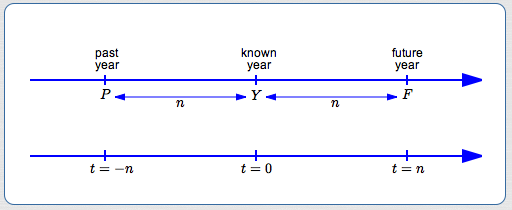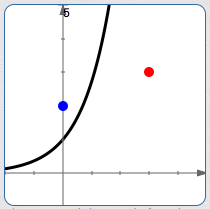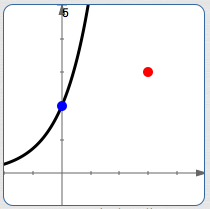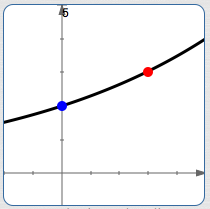﻿ Exponential Growth and Decay: Introduction

# EXPONENTIAL GROWTH AND DECAY: INTRODUCTION

by Dr. Carol JVF Burns (website creator)
Follow along with the highlighted text while you listen!
• PRACTICE (online exercises and printable worksheets)

Exponential functions are of the form $\,y = b^t\,$ for $\,b > 0\,$ and $\,b\ne 1\,.$
For $\,b > 1\,$ they are increasing, and are called exponential growth functions.
For $\,0 < b < 1\,$ they are decreasing, and are called exponential decay functions.

All exponential functions share common behavior:
when the input changes by a fixed amount, the output gets multiplied by a scaling factor.

It might be that every hour (the fixed input change), something doubles (gets multiplied by $\,2\,$).
Or, it might be that every three seconds (the fixed input change), something gets halved (multiplied by $\,\frac 12\,$).
In general, increasing exponential functions get big fast, and decreasing exponential functions get small fast.

A typical exponential growth/decay problem often has this flavor:

A population has a given initial size.
Later, it is bigger (or smaller).
Assuming exponential behavior, what is its size at some future time?

There are a couple things that can make exponential growth/decay problems seem tricky.
First of all, a ‘basic’ exponential function may need to be generalized, using both vertical and horizontal stretches.
Secondly, the generalized exponential functions go by lots of different names.
You might see a problem solved in different ways, and wonder ‘which is right’.
They're all right! Different people choose different names to work with!
These issues are discussed in this section.

## Choose $\,t = 0\,$

For exponential growth/decay problems, we call the input $\,t\,$ (for ‘time’) instead of $\,x\,.$
Populations change with time. Populations are a function of time.
The notation $\,P(t)\,$ denotes the size of a population at time $\,t\,.$

You always have an initial choice to make—what you call ‘time zero’—the ‘origin’ for time.
Some people choose the earliest time when a population is known to correspond to $\,t = 0\,.$
It doesn't matter, as long as you are consistent throughout the entire problem.

Suppose $\,t=0\,$ is chosen to correspond to time $\,Y\,$ (think ‘year’):Then, for $\,n > 0\,$: $\,t = n\,$ corresponds to $\,F = Y + n\,$ (future) $\,t = -n\,$ corresponds to $\,P = Y - n\,$ (past) Going the other direction, for $\,F > Y > P\,$: a future $\,F\,$ corresponds to positive time $\,t = F - Y\,$ a past $\,P\,$ corresponds to negative time $\,t = P - Y\,$

## Generalizing $\,y = b^t\,$ to Handle Typical Exponential Growth/Decay Problems

Pick any allowable value of $\,b\,.$ The sketches below assume $\,b > 1\,,$ but the discussion holds for any $\,b > 0\,,$ $\,b\ne 1\,.$A typical exponential function, $\,y = b^t\,.$ It may not have the correct initial size (the blue point). It may not have the correct second known size (the red point).Do a vertical scaling to get the correct initial size: $y = P_{\,0}\,b^t$Do a horizontal scaling to get the correct second known size: $y = P_{\,0}\,b^{st}$

The exponential function $\,P(t) = b^t\,$ is too restrictive for exponential growth/decay problems.
We need to allow for any initial population size. (The ‘initial population’ is the size at $\,t = 0\,.$ )
However, $\,P(0) = b^0 \ \overset{\text{always}}{=}\ 1\,.$ We don't always have an initial population of size $\,1\,$!

To solve this problem, do a vertical scaling of the basic exponential function, giving $\,P(t) = P_{\,0}\,b^t\,.$
With this generalization, $\,P(0) = P_{\,0}\, b^0 = P_{\,0}(1) = P_{\,0}\,,$ so $\,P_{\,0}\,$ is the desired initial population size.
(You can read ‘$\,P_{\,0}\,$’ as ‘$\,P\,$ sub zero’ or ‘$\,P\,$ naught’.)

We also need the curve to pass through a second point, which describes the population at some known future or past time.
A horizontal scaling accomplishes this:   $\,P(t) = P_{\,0}\, b^{st}\,$

## Checking that the Generalized Exponential Function Still Behaves As Expected

It's worthwhile to check that the generalized exponential function, $\,P(t) = P_{\,0}\, b^{st}\,,$ still has the property mentioned above:
when the input changes by a fixed amount, the output gets multiplied by a scaling factor.

\begin{alignat}{2} \cssId{s66}{P(t+\Delta t)} &\cssId{s67}{= P_{\,0} \, b^{s(t+\Delta t)}}&&\cssId{s68}{\text{definition of function \,P\,}}\cr\cr &\cssId{s69}{= P_{\,0} \, b^{(st+s\Delta t)}}&&\cssId{s70}{\text{distributive law}}\cr\cr &\cssId{s71}{= P_{\,0} \, b^{st}\, b^{s\Delta t}}&&\cssId{s72}{\text{exponent law}}\cr\cr &\cssId{s73}{= \bigl( P_{\,0\,} b^{st}\bigr) \cdot \bigl(b^{s\Delta t}\bigr)}\qquad &&\cssId{s74}{\text{re-group}}\cr\cr &\cssId{s75}{= P(t) \cdot \bigl(b^{s\Delta t}\bigr)}\qquad &&\cssId{s76}{\text{definition of \,P(t)\,}}\cr\cr \end{alignat}

In this case, the scaling factor is $\,b^{s\Delta t}\,,$ which depends on three things:

• the base of the exponential function ($\,b\,$)
• the horizontal scaling factor ($\,s\,$)
• how much the inputs are changing by ($\,\Delta t\,$)

## Any Generalized Exponential Function Can Be Written With Any Allowable Base

Let $\,b\,,$ $\,k\,$ and $\,s\,$ be the current base, vertical scaling factor, and horizontal scaling factor for a generalized exponential function: $$\cssId{s83}{y = kb^{st}}$$ You've decided you don't want base $\,b\,.$ You want a different base $\,B\,$ instead.
This can be easily done! Let $\,y = KB^{St}\,$ denote the new function, with the desired base.
Notice that we're using lowercase letters for the old function ($\,b\,,$ $\,k\,,$ $\,s\,$), and uppercase letters for the new function ($\,B\,,$ $\,K\,,$ $\,S\,$).

• We want $kb^{st} = KB^{St}$ to be true for all values of $\,t\,.$
• In particular, it must be true when $\,t = 0\,.$
Substituting $\,0\,$ for $\,t\,$ gives $\,kb^0 = KB^0\,,$ so that $\,k = K\,.$
The vertical scaling factor stays the same!
• Now we have $\,kb^{st} = kB^{St}\,.$
Divide both sides by $\,k\,,$ giving $\,b^{st} = B^{St}\,.$
• To get to the exponents, take logs of both sides.
Any log will do, so choose the natural logarithm:
$\ln b^{st} = \ln B^{St}$
• Bring down the exponents:
$st \ln b = St\ln B$
• Divide both sides by $\,t\,$:
$s\ln b = S\ln B$
• Solve for $\,S\,$:
$\displaystyle S = s\cdot\frac{\ln b}{\ln B}$

Here's a quick example to show how easy it is!
Initial function: $\,y = 10\cdot 5^{3t}\,$   ($\,k = 10\,,$ $\,b = 5\,,$ $\,s = 3\,$)
New desired base: $\,7\,$   ($\,B = 7\,$).

The $\,10\,$ stays the same ($\,K = k\,$).   The new horizontal scaling factor is $\,\displaystyle S = s\cdot\frac{\ln b}{\ln B} = 3\cdot \frac{\ln 5}{\ln 7}\,.$
The new function is therefore $\,y = K\cdot B^{St} = 10\cdot 7^{3t(\ln 5)/(\ln 7)}\,.$

Don't take my word for it!
Hop up to wolframalpha.com and cut-and-paste:

plot y = 10 * 5^{3t}, y = 10 * 7^{3t ln(5)/ln(7)}

You'll only see one curve, because they're exactly the same!

So, any base can be written as any other base. Exponential functions have lots of different names!
The point is this: when solving exponential growth/decay problems, you can use any (allowable) base that you want.
Having said this, though, there is a strongly-favored base that is usually used $\ldots$

## Since Any Base Can Be Used, Most People Use Base $\,\text{e}\,$

Since any base can be used when solving exponential growth/decay problems, most people use base $\,\text{e}\,.$
Why? Why would someone want to use an irrational number as a base, instead of a ‘simple’ number like (say) $\,2\,$?

The answer is that the exponential function with base $\,\text{e}\,$ has calculus properties that make it easier to work with than any other exponential function.
Also, when the base is $\,\text{e}\,,$ the horizontal scaling constant takes on special significance, and is given a special name—the relative growth rate.
More on this in the next section!

EXPONENTIAL GROWTH/DECAY solving exponential growth/decay problems
To model exponential growth or decay, most people use the function $$\cssId{s136}{P(t) = P_{\,0}\,{\text{e}}^{rt}}$$ where:
• $P(t) = \text{the population at time$\,t\,$}$
• $P_{\,0} = \text{the initial population} = \text{the population at$\,t = 0\,$}$
• The constant $\,r\,$ is called the relative growth rate.
(The relative growth rate is also called the exponential growth rate or continuous growth rate.)
• If $\,r > 0\,$ then $\,P_{\,0}\,{\text{e}}^{rt}\,$ is an increasing function; this is exponential growth.
• If $\,r < 0\,$ then $\,P_{\,0}\,{\text{e}}^{rt}\,$ is a decreasing function; this is exponential decay.
Master the ideas from this section